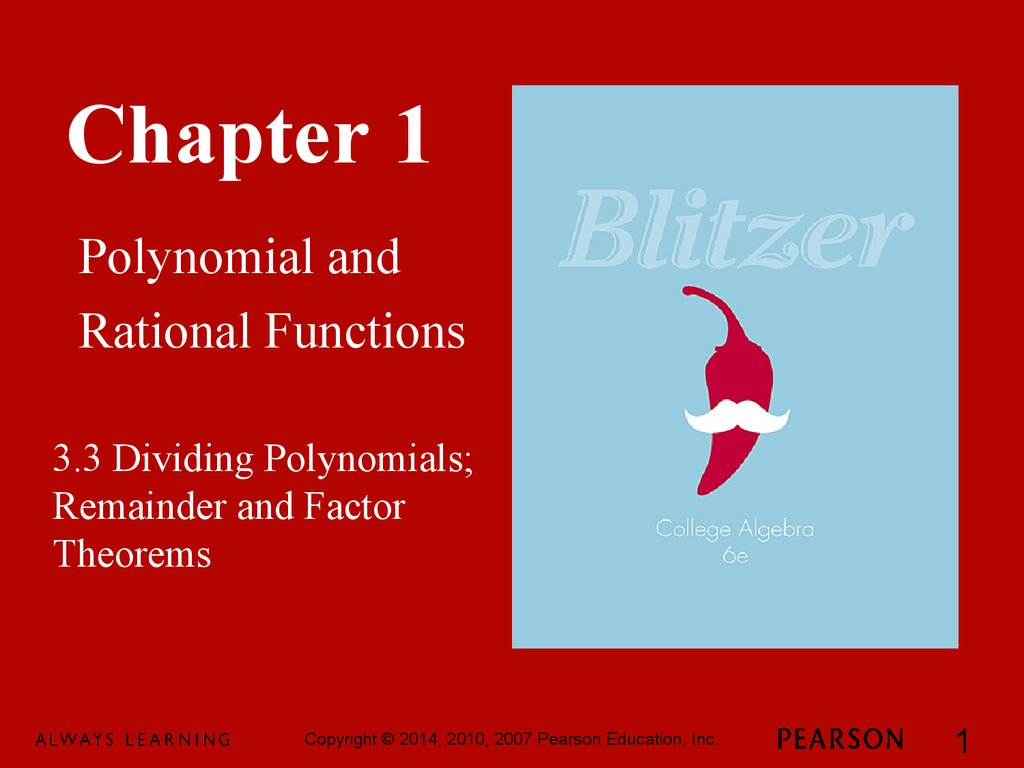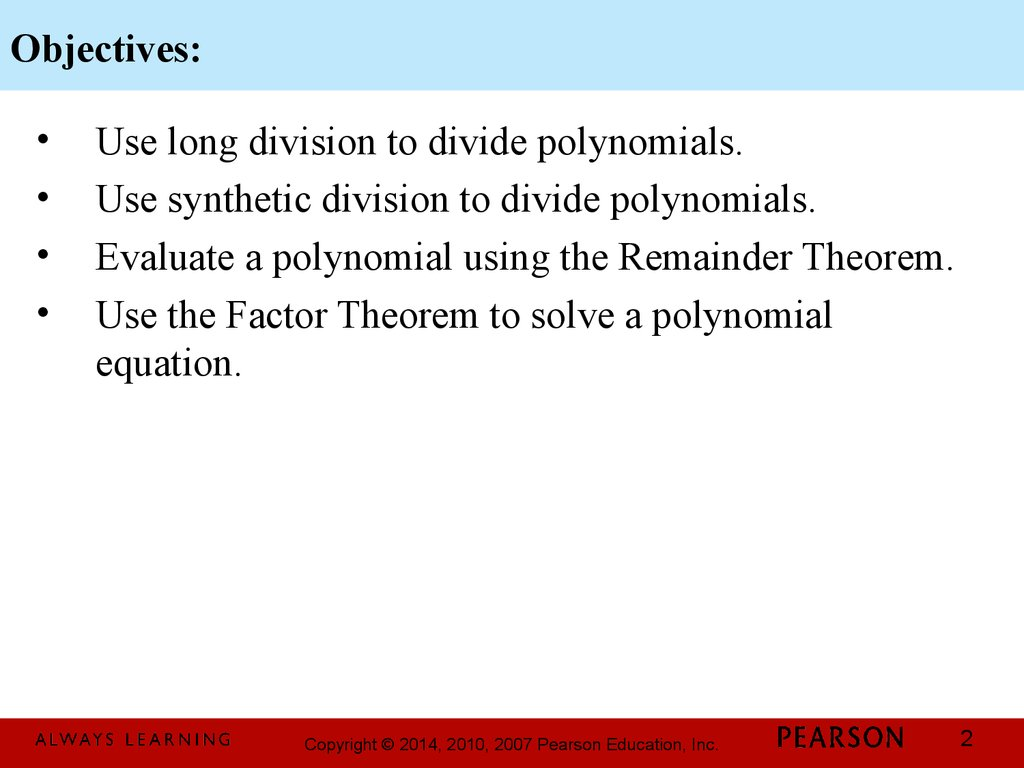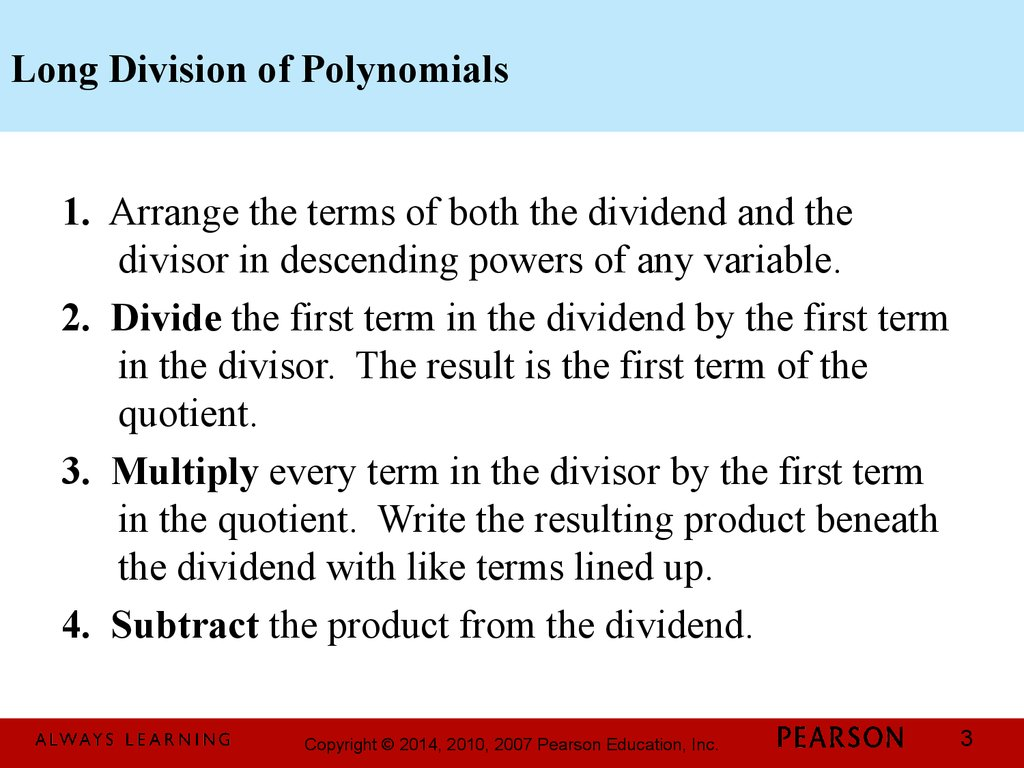# Chapter 1. Polynomial and Rational Functions. 3.3. Dividing Polynomials; Remainder and Factor Theorems

## 1.

Chapter 1
Polynomial and
Rational Functions
3.3 Dividing Polynomials;
Remainder and Factor
Theorems
1

## 2. Objectives:

Use long division to divide polynomials.
Use synthetic division to divide polynomials.
Evaluate a polynomial using the Remainder Theorem.
Use the Factor Theorem to solve a polynomial
equation.
2

## 3. Long Division of Polynomials

1. Arrange the terms of both the dividend and the
divisor in descending powers of any variable.
2. Divide the first term in the dividend by the first term
in the divisor. The result is the first term of the
quotient.
3. Multiply every term in the divisor by the first term
in the quotient. Write the resulting product beneath
the dividend with like terms lined up.
4. Subtract the product from the dividend.
3

## 4. Long Division of Polynomials (continued)

5. Bring down the next term in the original dividend
and write it next to the remainder to form a new
dividend.
6. Use this new expression as the dividend and repeat
this process until the remainder can no longer be
divided. This will occur when the degree of the
remainder (the highest exponent on a variable in the
remainder) is less than the degree of the divisor.
4

## 5. The Division Algorithm

If f(x) and d(x) are polynomials, with d ( x) ¹ 0 the
degree of d(x) is less than or equal to the degree of f(x) ,
then there exist unique polynomials q(x) and r(x) such
that
f ( x) = d ( x )gq ( x) + r ( x )
The remainder, r(x), equals 0 or it is of degree less than
the degree of d(x). If r(x) = 0, we say that d(x) divides
evenly into f(x) and that d(x) and q(x) are factors of f(x).
5

## 6. Example: Long Division of Polynomials

Divide 7 - 11x - 3 x 2 + 2 x 3 by x - 3
We begin by writing the dividend in descending powers
of x
7 - 11x - 3 x 2 + 2 x 3 = 2 x3 - 3 x 2 - 11x + 7
6

## 7. Example: Long Division of Polynomials (continued)

Divide 2 x 3 - 3 x 2 - 11x + 7 by x - 3
22x
x 22 + 3 x - 2
x - 3 2 x 3 - 3 x 2 - 11x + 7
-2 x3 + 6x2
3 x 2 - 11x
-3 x 2 -+ 9 x
-2 x + 7
-2 x +6
1
The quotient is
1
2 x + 3x - 2 +
x-3
2
7

## 8. Synthetic Division

1. Arrange the polynomial in descending powers, with a
0 coefficient for any missing term.
2. Write c for the divisor, x – c. To the right, write the
coefficients of the dividend.
3. Write the leading coefficient of the dividend on the
bottom row.
4. Multiply c times the value just written on the bottom
row. Write the product in the next column in the
second row.
8

## 9. Synthetic Division (continued)

5. Add the values in this new column, writing the sum
in the bottom row.
6. Repeat this series of multiplications and additions
until all columns are filled in.
7. Use the numbers in the last row to write the quotient,
plus the remainder above the divisor. The degree of
the first term of the quotient is one less than the
degree of the first term of the dividend. The final
value in this row is the remainder.
9

## 10. Example: Using Synthetic Division

Use synthetic division to divide x - 7 x - 6 by x + 2
The divisor must be in form x – c. Thus, we write x + 2
as x – (–2). This means that c = –2. Writing a 0
coefficient for the missing x2 term in the dividend, we
can express the division as follows:
3
x - (-2) x 3 + 0 x 2 - 7 x - 6
Now we are ready to perform the synthetic division.
10

## 11. Synthetic Division (continued)

Use synthetic division to divide x - 7 x - 6 by x + 2.
3
-2 1
0 -7 -6
--22 44 6
11 --22 -3 0
2
x
The quotient is - 2 x - 3.
11

## 12. The Remainder Theorem

If the polynomial f(x) is divided by x – c, then the
remainder is f(x).
12

## 13. Example: Using the Remainder Theorem to Evaluate a Polynomial Function

Given f ( x) = 3 x + 4 x - 5 x + 3 use the Remainder
Theorem to find f(–4).
We use synthetic division to divide.
3
2
-4 3 +4 - 5 + 3
-12
¯ 12 +32 -108
3 -8 -27 -105
The remainder, –105, is the value of f(–4). Thus,
f(–4) = –105
13

## 14. The Factor Theorem

Let f(x) be a polynomial.
a. If f(x) = 0, then x – c is a factor of f(x).
b. If x – c is a factor of f(x), then f(c) = 0
14

## 15. Example: Using the Factor Theorem

Solve the equation 15 x 3 + 14 x 2 - 3x - 2 = 0 given that –
3
2
1 is a zero of f ( x) = 15 x + 14 x - 3 x - 2.
We are given that –1 is a zero of
f ( x) = 15 x 3 + 14 x 2 - 3x - 2. This means that f(–1) = 0.
Because f(–1) = 0, the Factor Theorem tells us that x + 1
is a factor of f(x). We’ll use synthetic division to divide
f(x) by x + 1.
15

## 16. Example: Using the Factor Theorem

Solve the equation 15 x 3 + 14 x 2 - 3x - 2 = 0 given that –
3
2
1 is a zero of f ( x) = 15 x + 14 x - 3 x - 2.
We’ll use synthetic division to divide f(x) by x + 1.
-1 15 +14 -3 -2
¯ -15
15 +1 +2
15 -1 -2 0
(15 x + 14 x - 3 x - 2) ¸ ( x + 1) = 15 x - x - 2
3
2
2
This means that
(15 x 3 + 14 x 2 - 3x - 2) = ( x + 1)(15 x 2 - x - 2)
16

## 17. Example: Using the Factor Theorem (continued)

Solve the equation 15 x 3 + 14 x 2 - 3x - 2 = 0 given that –
3
2
1 is a zero of f ( x) = 15 x + 14 x - 3 x - 2.
(15 x 3 + 14 x 2 - 3 x - 2) = ( x + 1)(15 x 2 - x - 2)
(15 x 3 + 14 x 2 - 3x - 2) = ( x + 1)(3 x + 1)(5 x - 2)
x + 1 = 0 ® x = -1
1
3x + 1 = 0 ® x = 3
2
5x - 2 = 0 ® x =
5
The solution set is
1 2
-1, - ,
3 5
{